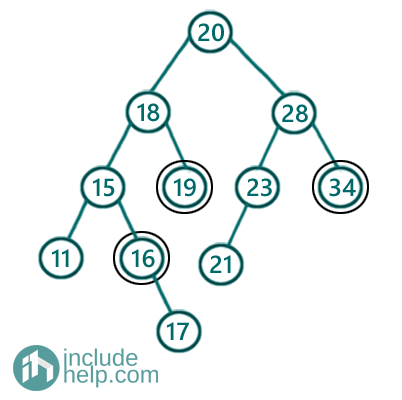# Distance between two nodes in a BST

In this article, we are going to see how to find distances between two nodes in a Binary Search Tree?
Submitted by Radib Kar, on November 02, 2020

In this article, we are going to see given two nodes of a BST, how can we find the distance b/w them. In our past article (Minimum distance between two given nodes of a Binary Tree), we have seen that in case of a normal binary tree we need to find the LCA of the two nodes. Here also, in a Binary Search Tree, we can do the same, but not in a similar way. Rather, we will take advantage of the BST properties.

For example,

Say the tree is like below:If two nodes are 16 & 34 then their distance is 5 and if two given nodes are 16 & 19, then their distance is 3.

So, first thing is to observe that,

1. two given nodes can lie in different subtrees, i.e., one node will lie in the left subtree and another in the right subtree. Which is the case for 16 & 34. In that case, our LCA is the root and total distance would be= distance from the root to 16 + distance from the root to 34
2. Two given node can lie in the same subtree which is the case for 16 & 19. In this case, we need to reach the LCA first, and then it will be similar as of the first case. Total distance would be the distance from LCA to node1 + distance from LCA to node2

But in the case of BST, we don't need to check for LCA separately.
We can check whether, both the node values are greater than the current root or not. If both are greater than the current root, then both of the nodes fall in the right subtree, thus we simply recur for the right subtree.

If both of the node values are less than the current root, then both of the nodes fall in the left subtree, thus we simply recur for the left subtree.

Otherwise if above are not the cases, that means the given two nodes fall on different subtrees rooted by the current root. Thus the current root is the LCA and we can find the distances from this current root to that designated nodes individually and sum up to find minimum distances between two given nodes in a Binary Search Tree.

Below is the detailed C++ implementation.

```#include <bits/stdc++.h>
using namespace std;

// tree node is defined
class TreeNode {
public:
int val;
TreeNode* left;
TreeNode* right;
TreeNode(int data)
{
val = data;
left = NULL;
right = NULL;
}
};

//distance b/w a node and the root of the subtree
int findDistance(TreeNode* root, int v)
{
int dist = 0;
TreeNode* cur = root;
while (cur) {
if (cur->val == v)
return dist;
else {
dist++;
if (cur->val > v)
cur = cur->left;
else
cur = cur->right;
}
}
return 0;
}

//function that finds distance b/w two nodes in a BST
int distanceBWNodes(TreeNode* root, int v1, int v2)
{
//null tree
if (!root)
return 0;
//same node
if (v1 == v2)
return 0;

if (root->val < v1 && root->val < v2) {
return distanceBWNodes(root->right, v1, v2);
}
else if (root->val > v1 && root->val > v2) {
return distanceBWNodes(root->left, v1, v2);
}
else {
return findDistance(root, v1) + findDistance(root, v2);
}
}

int main()
{
TreeNode* root = new TreeNode(20);
root->left = new TreeNode(18);
root->right = new TreeNode(28);
root->left->left = new TreeNode(15);
root->left->right = new TreeNode(19);
root->right->left = new TreeNode(23);
root->right->right = new TreeNode(34);
root->left->left->left = new TreeNode(11);
root->left->left->right = new TreeNode(16);
root->left->left->right->right = new TreeNode(17);
root->right->left->left = new TreeNode(21);

cout << "Distances B/w nodes 16 & 19 is: " << distanceBWNodes(root, 16, 19) << endl;
cout << "Distances B/w nodes 16 & 34 is: " << distanceBWNodes(root, 16, 34) << endl;

return 0;
}
```

Output:

```Distances B/w nodes 16 & 19 is: 3
Distances B/w nodes 16 & 34 is: 5
```

Since, the idea is pretty intuitive and the code itself given an illustration we are skipping the dry run. In case, if you need any help, please comment below to let us know.